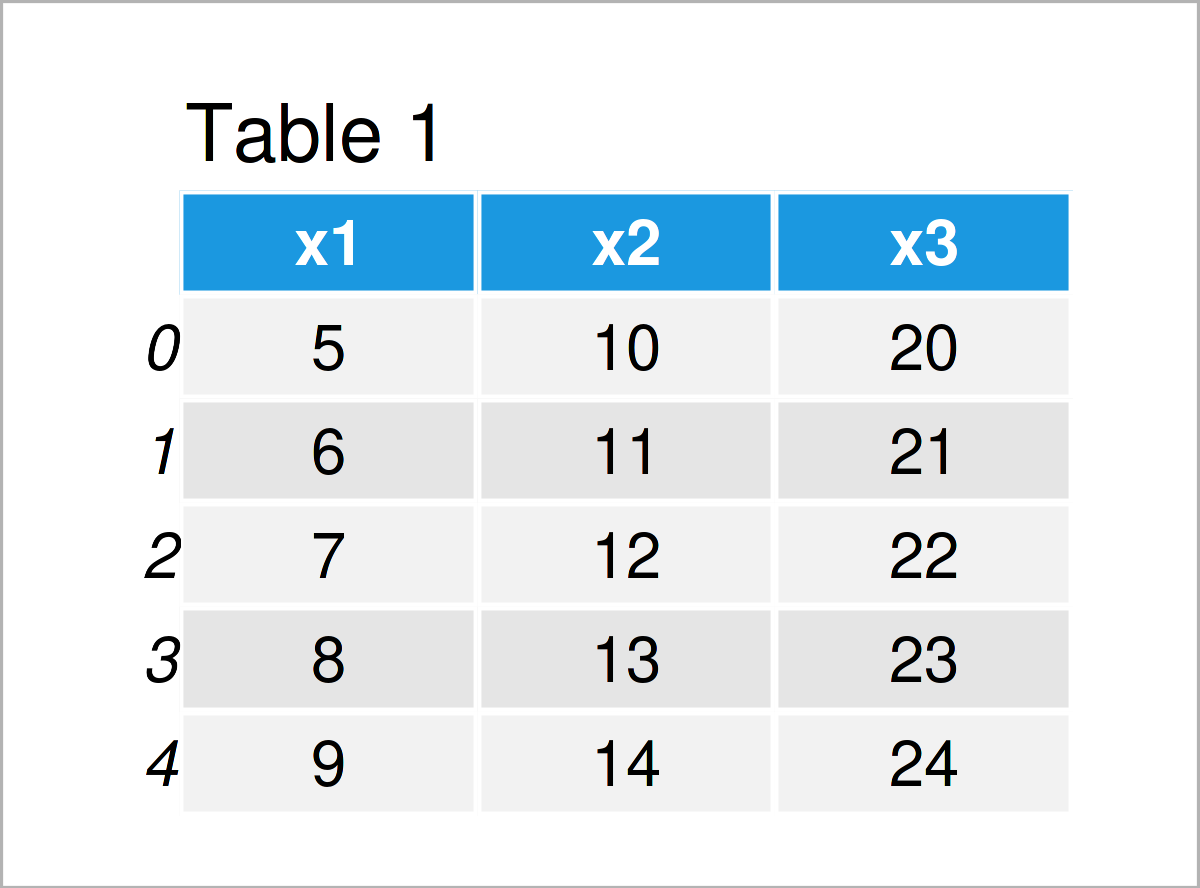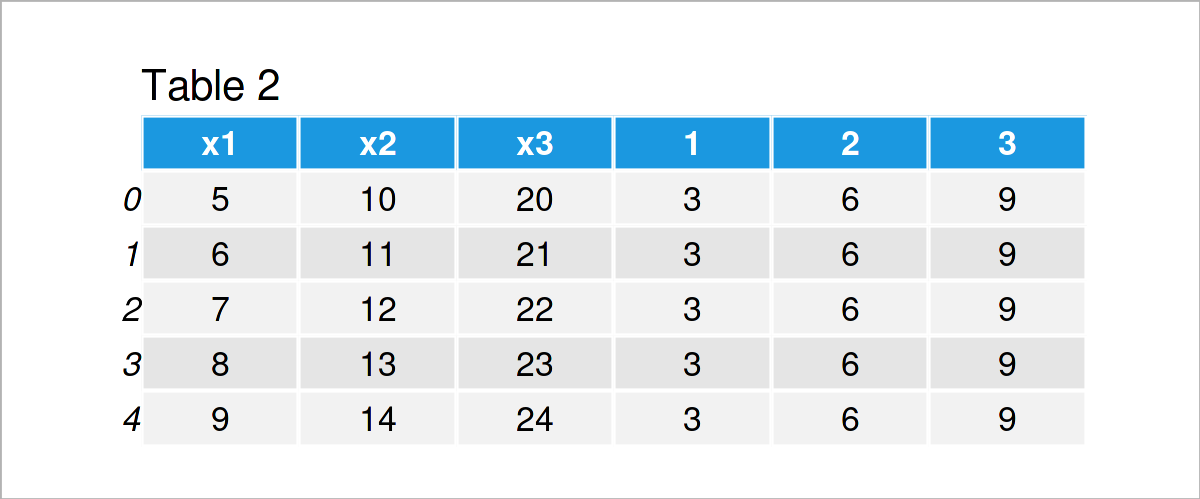# Append Columns to pandas DataFrame in Loop in Python (Example)

This tutorial demonstrates how to add new columns to a pandas DataFrame within a for loop in Python programming.

The article will contain one example for the addition of new variables to a pandas DataFrame within a for loop. To be more specific, the post is structured as follows:

Let’s dive right into the example…

## Example Data & Libraries

We first have to import the pandas library, if we want to use the corresponding functions:

`import pandas as pd                        # Load pandas`

In addition, have a look at the following example data:

```data = pd.DataFrame({'x1':range(5, 10),    # Create pandas DataFrame
'x2':range(10, 15),
'x3':range(20, 25)})
print(data)                                # Print pandas DataFrame```Have a look at the table that got returned after executing the previously shown Python programming code. It shows that our example pandas DataFrame is constructed of five data points and three columns.

## Example: Append Columns to pandas DataFrame within for Loop

In this example, I’ll illustrate how to use a for loop to append new variables to a pandas DataFrame in Python.

Have a look at the Python syntax below. It shows a for loop that consists of two lines.

The first line specifies that we want to iterate over a range from 1 to 4.

The second line specifies what we want to do in this loop, i.e. in each iteration we want to add a new column containing the iterator i times the value three. The variable name of this new column should be called like the iterator.

Let’s do this:

```for i in range(1, 4):                      # Append columns within for loop
data[i] = i * 3
print(data)                                # Print updated DataFrame```Table 2 shows the output of the previous code: We have extended our example data set by three new columns.

## Video & Further Resources

I have recently published a video on my YouTube channel, which illustrates the Python programming code of this article. You can find the video below.

Additionally, you could read the other posts on this homepage. A selection of articles is shown below.